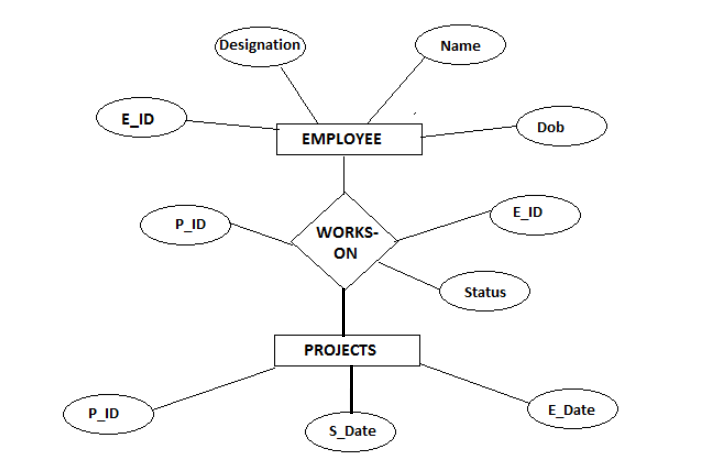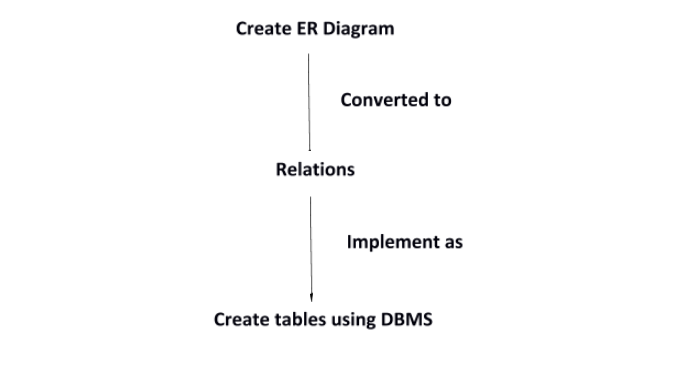# Converting E-R model into relational model

A given ER model can be converted into Relational model. A Relational model includes Relations, Tuples, Attributes, Keys, and Foreign keys.

• Relation is a table made from tuples.

• A Tuple is a row of data.

• An Attribute is a characteristic of the relation.

There is a direct mapping between ER model and Relational model.

Consider the following exampleRules of converting ER model to Relational Model-

• Entity type is converted to a Relation table.

• 1:1 or 1: N relationship type is converted to foreign key.

• M: N relationship type is converted to a relation with two foreign key.

• Simple attribute converted to an attribute.

• Value set converted to a domain.

• Key attribute converted to a primary key.

Now for the above example we can create three relations-

• Employee

• Works_On

• Projects

Transform attributes to fields-

• Employee will have E_ID, Name, Designation and Dob.

• Works_On will have E_ID, Status and P_ID.

• Projects will have P_ID, S_Date and E_Date.

Now we can create tables in DBMS.

Overall transformation summary is −